This notebook is an element of the risk-engineering.org courseware. It can be distributed under the terms of the Creative Commons Attribution-ShareAlike licence.

Author: Eric Marsden eric.marsden@risk-engineering.org

In this notebook, we illustrate features of the NumPy, Pandas and statsmodels libraries features for building linear regression models in Python. Consult the accompanying course materials for background on linear regression analysis, some notes on when the technique is useful, and to download this content as a Jupyter/Python notebook.

# Linear regression analysis of combined cycle power plant data¶

In :
import numpy
import pandas
import matplotlib.pyplot as plt
import scipy.stats
plt.style.use("bmh")
%config InlineBackend.figure_formats=["png"]


We will analyze data from a combined cycle power plant to attempt to build a predictive model for output power. The data comes from the UCI machine learning repository.

http://archive.ics.uci.edu/ml/datasets/Combined+Cycle+Power+Plant

The dataset contains 9568 data points collected from a combined cycle power plant over 6 years, when power plant was under full load. A combined cycle power plant is composed of gas turbines, steam turbines and heat recovery steam generators. Electricity is generated by gas & steam turbines, which are combined in one cycle. Three ambient variables affect the performance of the gas turbine, and exhaust vacuum affects the performance of the steam turbine.

Data consists of hourly averages taken from various sensors located around the plant that record the ambient variables every second.

Let’s load it into Python and examine it using the pandas library. For convenience, we have unzipped the dataset and made it web accessible.

In :
data = pandas.read_csv("https://risk-engineering.org/static/data/CCPP.csv")

Out:
AT V AP RH PE
0 14.96 41.76 1024.07 73.17 463.26
1 25.18 62.96 1020.04 59.08 444.37
2 5.11 39.40 1012.16 92.14 488.56
3 20.86 57.32 1010.24 76.64 446.48
4 10.82 37.50 1009.23 96.62 473.90

The table below provides the meaning of the various columns.

Meaning Name Range
Ambient Temperature AT 1.81 – 37.11°C
Ambient Pressure AP 992.89 – 1033.30 millibar
Relative Humidity RH 25.56% – 100.16%
Exhaust Vacuum V 25.36 – 81.56 cm Hg
Net hourly electrical energy output PE 420.26 – 495.76 MW
In :
data.describe()

Out:
AT V AP RH PE
count 9568.000000 9568.000000 9568.000000 9568.000000 9568.000000
mean 19.651231 54.305804 1013.259078 73.308978 454.365009
std 7.452473 12.707893 5.938784 14.600269 17.066995
min 1.810000 25.360000 992.890000 25.560000 420.260000
25% 13.510000 41.740000 1009.100000 63.327500 439.750000
50% 20.345000 52.080000 1012.940000 74.975000 451.550000
75% 25.720000 66.540000 1017.260000 84.830000 468.430000
max 37.110000 81.560000 1033.300000 100.160000 495.760000

## Visualization¶

We can obtain a first impression of the dependency between variables by examining a multidimensional scatterplot.

In :
from pandas.plotting import scatter_matrix
scatter_matrix(data, diagonal="kde");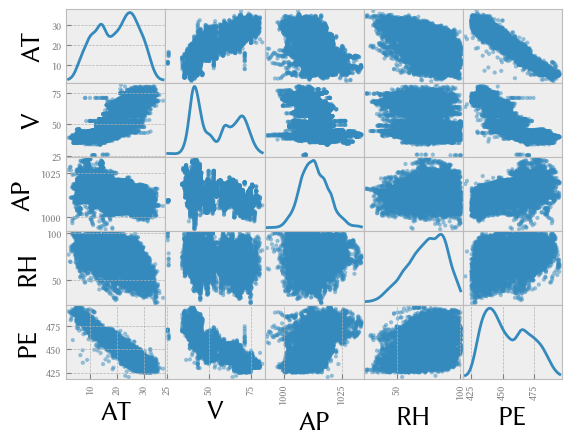In this matrix, the diagonal contains a plot of the distribution of each variable. We observe that:

• there is an approximately linear relationship between PE and the negative of AT

• there is an approximately linear relationship between PE and negative of V

We can also generate a 3D plot of the observations, which can sometimes help to interpret the data more easily. Here we plot PE as a function of AT and V.

In :
fig = plt.figure(figsize=(12, 8))
ax.scatter(data["AT"], data["V"], data["PE"])
ax.set_xlabel("AT")
ax.set_ylabel("V")
ax.set_zlabel("PE")
ax.set_facecolor("white")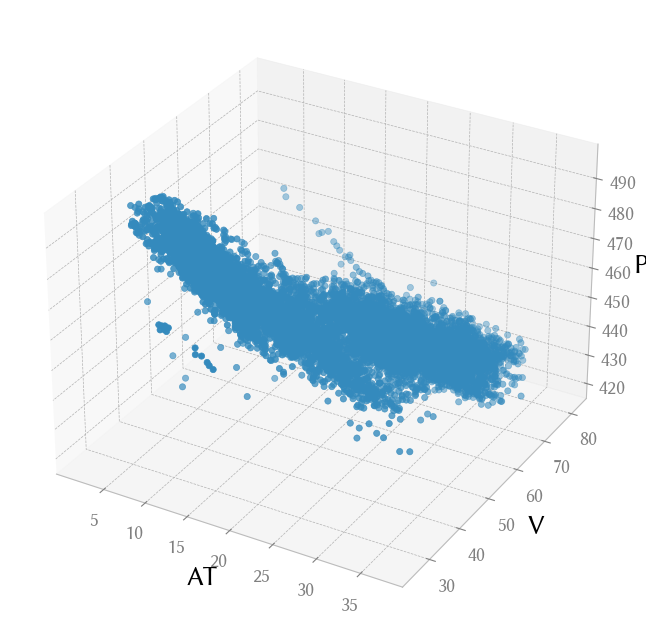## Prediction¶

We will created a fitted linear model using the formula API of the statsmodels library. We use all the observations except for PE as predictor variables in the multiple linear regression.

In :
import statsmodels.formula.api as smf

lm = smf.ols(formula="PE ~ AT + V + AP + RH", data=data).fit()
lm.params

Out:
Intercept    454.609274
AT            -1.977513
V             -0.233916
AP             0.062083
RH            -0.158054
dtype: float64

This means that the best formula to estimate output power as a function of AT, V, AP and RH is

$$PE = 451 . 067793 − 1 . 974731 AT − 0 . 234992 V + 0 . 065540 AP − 0 . 157598 RH$$

For any particular observation (values for the predictor variables), we can use the linear model to estimate the output variable PE.

In :
lm.predict(pandas.DataFrame({"AT": [9.48], "V": [44.71], "AP": [1019.12], "RH": [66.43]}))

Out:
0    478.174482
dtype: float64

The predicted output power for this combination of inputs is 478 MW (note that we report the result with the same number of significant figures as that in our inputs).

## Residuals plots¶

We check the residuals of each predictor variable for any pattern that might indicate that a linear model is not appropriate for this dataset.

In :
residuals = lm.predict(data) - data.PE
plt.plot(data.AT, residuals, ".", alpha=0.5)
plt.xlabel("AT")
plt.ylabel("Residual");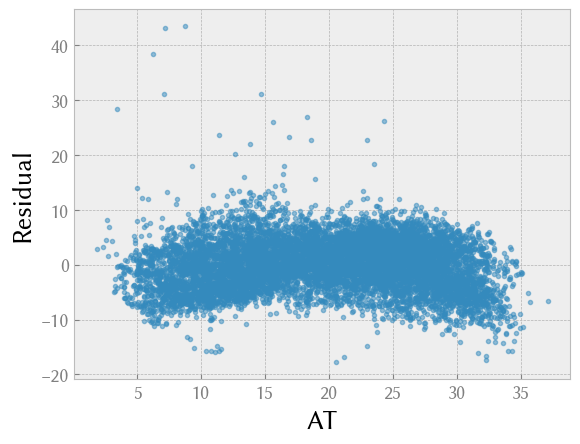In :
plt.plot(data.V, residuals, ".", alpha=0.5)
plt.xlabel("V")
plt.ylabel("Residual");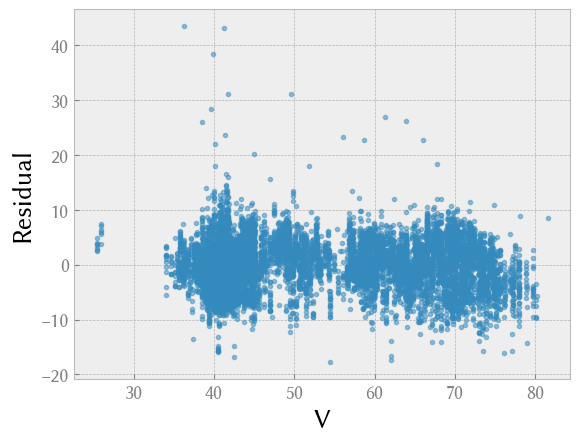In :
plt.plot(data.AP, residuals, ".", alpha=0.5)
plt.xlabel("AP")
plt.ylabel("Residual");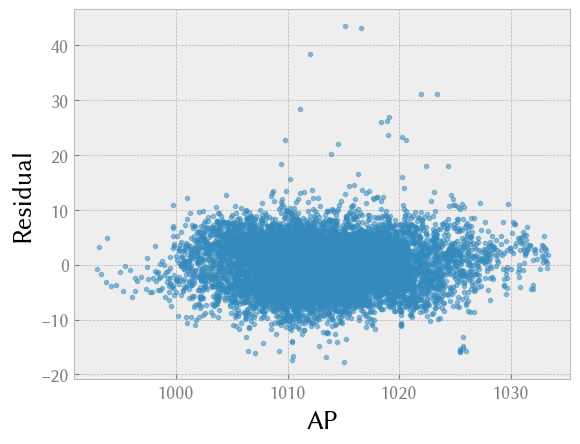In :
plt.plot(data.RH, residuals, ".", alpha=0.5)
plt.xlabel("RH")
plt.ylabel("Residual");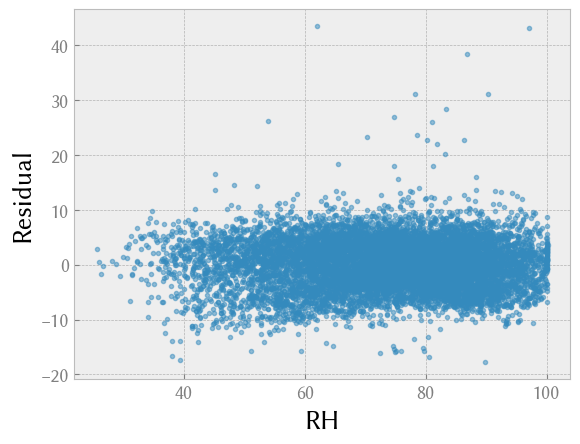Indeed, except for a minor quadratic shape to the residuals of variable AT (which we will ignore here), the residuals look random, without any systematic feature apparent that might indicate that our linear model is not appropriate for this data.

We also check that the variance of the residuals is normally distributed by plotting a histogram or a QQ plot of the residuals, as shown below.

In :
plt.hist(residuals, bins=40, alpha=0.5)
plt.title("Histogram of the residuals");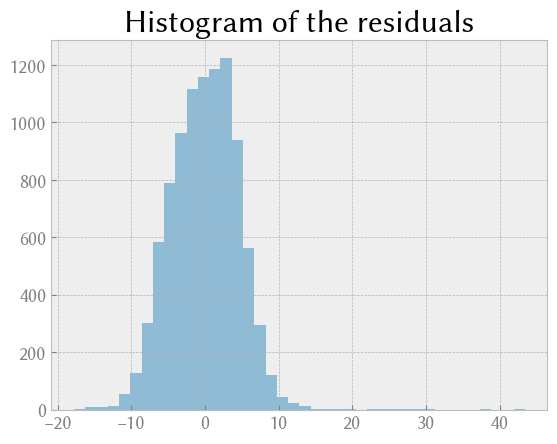In :
scipy.stats.probplot(residuals, dist=scipy.stats.norm, plot=plt.figure().add_subplot(111));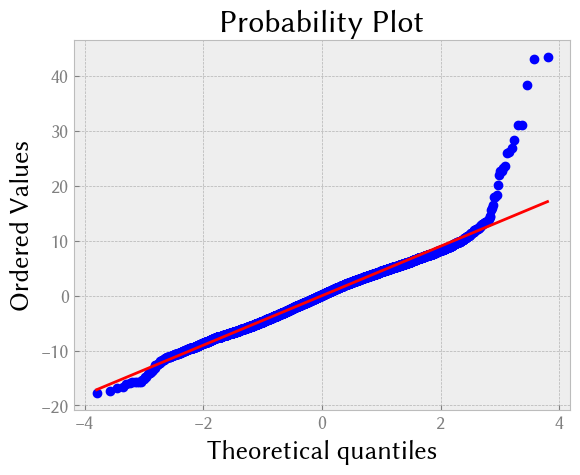## Goodness of fit¶

In :
lm.summary()

Out:
Dep. Variable: R-squared: PE 0.929 OLS 0.929 Least Squares 3.114e+04 Thu, 06 Jul 2023 0.00 15:20:19 -28088. 9568 5.619e+04 9563 5.622e+04 4 nonrobust
coef std err t P>|t| [0.025 0.975] 454.6093 9.749 46.634 0.000 435.500 473.718 -1.9775 0.015 -129.342 0.000 -2.007 -1.948 -0.2339 0.007 -32.122 0.000 -0.248 -0.220 0.0621 0.009 6.564 0.000 0.044 0.081 -0.1581 0.004 -37.918 0.000 -0.166 -0.150
 Omnibus: Durbin-Watson: 892.002 2.033 0 4086.78 -0.352 0 6.123 213000

Notes:
 Standard Errors assume that the covariance matrix of the errors is correctly specified.
 The condition number is large, 2.13e+05. This might indicate that there are
strong multicollinearity or other numerical problems.

The $R^2$ for this model is 0.929, which means that it explains roughly 93% of the variance of the output power. Interpretation of this number is dependent on the application (for instance, models used to understand health effects tend to have lower $R^2$ values than those used for physical models), but is quite satisfactory for this application.

In [ ]: